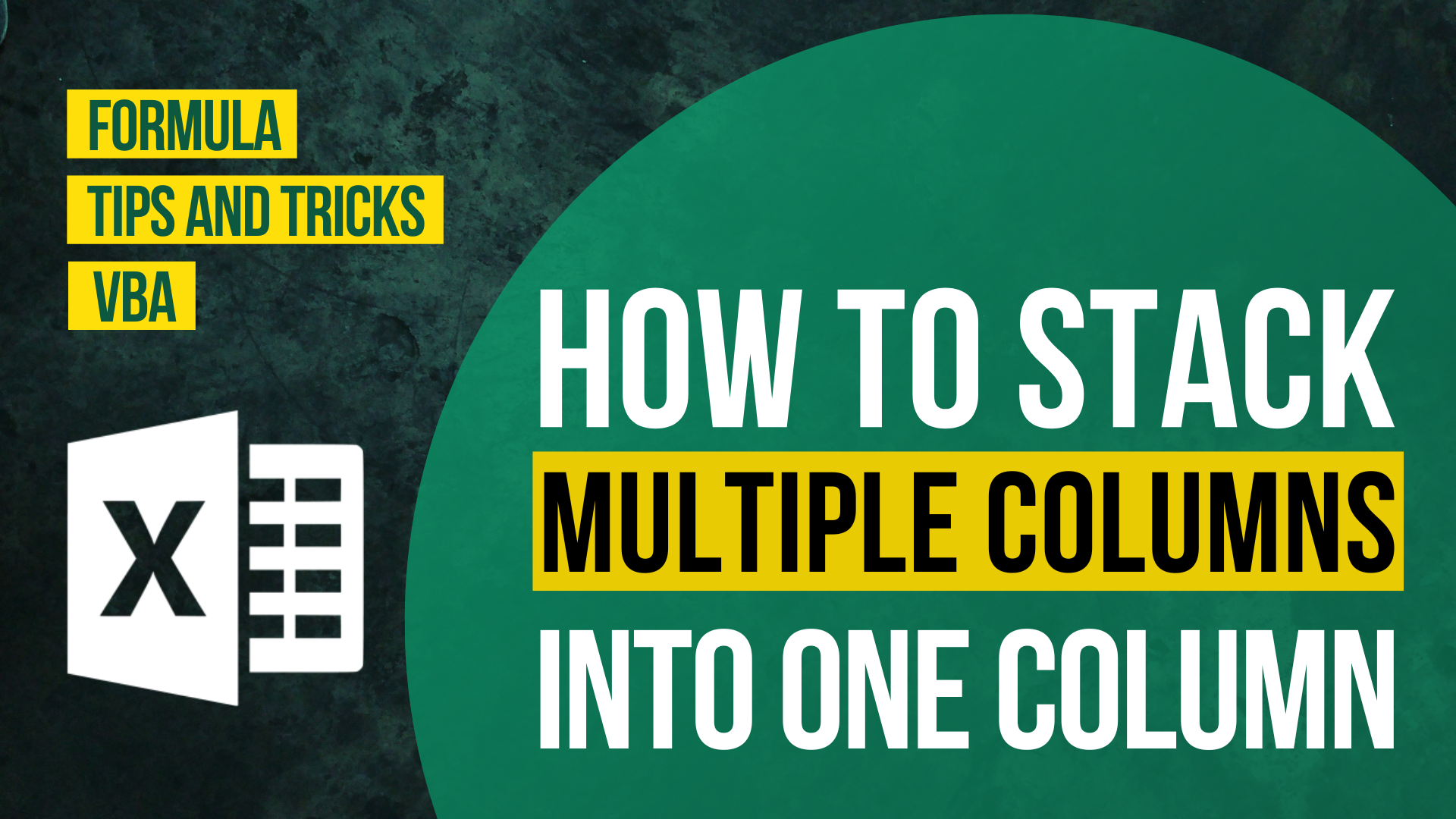# How to Display the Formula as Text in Excel?## Background

If you click into this article, you must be looking for a way to solve the below problems.

• How to get the formula displayed as text in Excel?
• How to display formula rather than result in Excel?
• How to Show Formulas in Excel Instead of the Values?

In general, there are two possible solutions to above-mentioned problem.

1. Show every cell as formulas
2. Display the formula of a cell in another cell

To show every cell as formulas, all you need is a shortcut.

To display the formula of one cell in another cell, I saw some Excel users trying to do it with CONCATENATE or tweaking the number format. Actually there is a function dedicated to solve these problems – FORMULATEXT function.

In this article, I will show you how to display the formula as text in Excel in the original cell using a shortcut and in a different cell FORMULATEXT function.

## Example

What we are trying to do here is to get Cell B5 display as formula rather than values.

## Option 1: To show every cell as formulas

To do this, all you need is a shortcut.

Here is the shortcut.

To apply the shortcut, just press any cell in the Excel sheet.

After applying the shortcut, cells contain formula will display its formula rather than the results.

Expected outcome of option 1

If you press the Ctrl ~ again, it will return to its original place – showing value instead of formula.

The shortcut is actually the shortcut of the button called “Show Formulas”, which is under the “Formulas” tab.

Limitations of “Show Formulas”

“Show Formulas” is designated for error checking/ formula inconsistency checking purpose.

Once you press the button, every single cell in the worksheet will be display its formula instead of values.

If you want it to display the result again, you have to press the button again.

However, each of those actions are applied to the whole worksheet (other worksheets in the workbook is not affected). You cannot apply the action towards one specific cell only.

Also, this shortcut only allows you to display the formula in the original cell. You cannot extract the formula in other cells by using this. If this is what you want, you should look into option 2.

## Option 2: FORMULATEXT function

### FormulaText function

To begin with, I would like to introduce FORMULATEXT function to you.

As suggested by its name, FORMULATEXT function returns a formula as a text string from a specified reference.

FORMULATEXT() Syntax:

`=FORMULATEXT(reference)`

To apply FORMULATEXT function, you simply have to put a cell reference into it.

Expected outcome of option 2

In the above example, the formula of Cell B5 is displayed in Cell D5.

The text displayed in Cell D5 is not the formula of Cell D5, but the value resulted from the formula.

Let me show you what actually is the formula of Cell D5.

Formula of Cell D5:

`=FORMULATEXT(B5)`

Limitations of “FORMULATEXT function”

To apply this function, you will need another cell. You can’t just display the formula in the original cell.

If you would like to display the formula in the original cell but you only want to do that for certain cells, then you should go for this.

This is a really simple solution.

All you need is to add an apostrophe before the equal sign.

The process of adding the apostrophe can be time-consuming and manual. Of course you can do it with CONCATENATE function but that will require more time and it’s no longer done within the same cell.How to Input Time Duration in ExcelHow to Remove Bullets/Numberings in Excel

Popular posts